View Solutions

1. Explain why induced emf is sometimes also called back emf.

2. Will a current be always induced whenever there is change in magnetic flux?

3.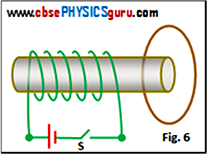4. Can electric current be induced in a closed loop held stationary in the very strong magnetic field between the north and south poles of two permanent fixed magnets?

5. A closed loop enters normally to the constant electric field between the plates of a large capacitor, moves wholly inside the field and then comes out of the field. Is a current induced in the loop
1. when it is entering the field
2. when it is wholly inside the region between the capacitor plates
3. when it is leaving the field?

6. A conducting loop is placed near a solenoid as shown in figure 6. Find the direction of the induced current in the loop
1. at the instant the switch S is closed,
2. after the switch has been closed for a long time,
3. at the instant the switch is opened.

7. A rectangular loop and a circular loop are moving out of a uniform magnetic field with a constant velocity v as shown in figure 7. Explain the variation of induced emf during the passage out of the field? The field is normal to the loops.

8.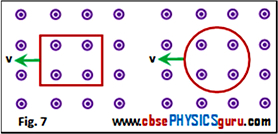9. A bar magnet falls vertically through a horizontal aluminum ring with its length along the axis of the ring as shown in fig. 5 (a).What will the acceleration of the falling magnet as compared to the acceleration due to gravity?

10. An astronaut on space journey reaches an unknown planet. He has only a sensitive galvanometer and a coil with him. Can he detect the presence of the magnetic field on the planet? If yes, how?

11. Explain why are the coils of resistance box made up of doubled up insulated wire?

12. A magnet falls freely through a circular loop having a cut in it. What will be acceleration of the magnet?

13. A loop of wire is removed from the magnetic field (a) rapidly (b) slowly. Compare the work done in (a) and (b)?

14. Is induced electric field a non conservative field?

15. A copper plate is first pushed into a magnetic field and then pulled out of it. In which motion, there will be an opposing force acting on the plate?

16.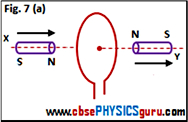17. Two identical circular coaxial coils carry equal currents in the same direction. If the coils are brought closer, what will happen to the currents in them?

18. A circular conducting coil moves normal to the electric field between the plates of a capacitor. Will there be a current induced in the coil when it moves:
1. wholly inside the plates of the capacitor
2. partially outside the plates of the capacitor?

19.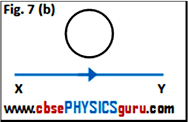20. Two identical magnets X and Y move relative to a fixed coil with same speed as shown in the figure 7 (a). What is the induced emf?

21. The electric current flowing in a wire in the direction X to Y is increasing. What is direction of the induced current in the conducting ring kept above the wire with its plane being parallel to the wire as shown in figure 7 (b)?

22.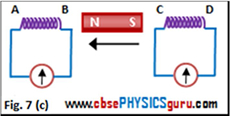23. What is the magnitude of the induced current in the circular ring of radius r as shown in figure 7 (b), if the straight wire XY carries a steady current of magnitude I ampere?

24. A magnet is moved in the direction indicated by the arrow between two coils AB and CD as shown in figure 7 (c). Suggest the direction of current in each coil.

25. A vertical metal rod falls freely. Will there be an emf induced between the ends of the rod?

CBSE Electromagnetic Induction ( With Hint / Solution)
Class XII (By Mr. Ashis Kumar Satapathy)
email - [email protected]

Electromagnetic Induction